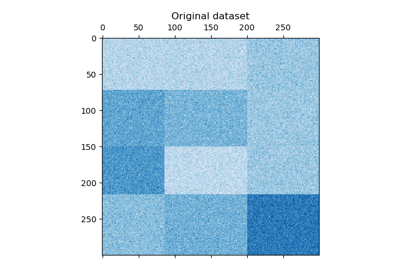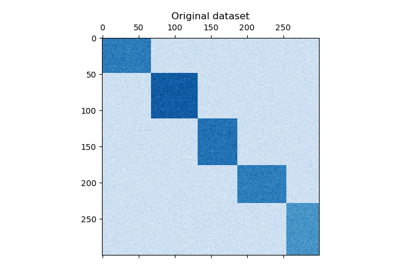# sklearn.metrics.consensus_score¶

sklearn.metrics.consensus_score(a, b, *, similarity='jaccard')[source]

The similarity of two sets of biclusters.

Similarity between individual biclusters is computed. Then the best matching between sets is found using the Hungarian algorithm. The final score is the sum of similarities divided by the size of the larger set.

Read more in the User Guide.

Parameters:
atuple (rows, columns)

Tuple of row and column indicators for a set of biclusters.

btuple (rows, columns)

Another set of biclusters like a.

similarity‘jaccard’ or callable, default=’jaccard’

May be the string “jaccard” to use the Jaccard coefficient, or any function that takes four arguments, each of which is a 1d indicator vector: (a_rows, a_columns, b_rows, b_columns).

Returns:
consensus_scorefloat

Consensus score, a non-negative value, sum of similarities divided by size of larger set.

References

## Examples using sklearn.metrics.consensus_score¶A demo of the Spectral Biclustering algorithm

A demo of the Spectral Biclustering algorithmA demo of the Spectral Co-Clustering algorithm

A demo of the Spectral Co-Clustering algorithm